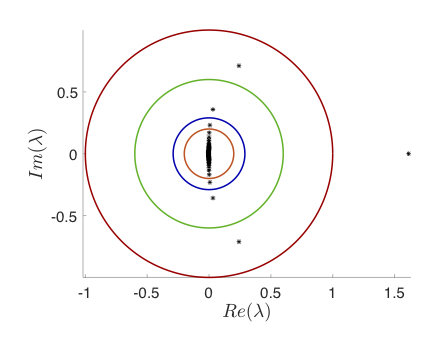### A functional analytic approach to validated numerics for eigenvalues of delay equations

Jean-Philippe Lessard and Jason Mireles James

This work develops validated numerical methods for linear stability analysis at an equilibrium solution of a system of delay differential equations (DDEs). The case of a single constant delay is considered. The method downplays the role of the scalar transcendental characteristic equation in favor of a functional analytic approach exploiting the strengths of numerical linear algebra/techniques of scientific computing. The idea is to consider an equivalent implicitly defined discrete time dynamical system which is projected onto a countable basis of Chebyshev series coefficients. The projected problem reduces to questions about certain sparse infinite matrices, which are well approximated by N x N matrices for large enough N. We develop the appropriate truncation error bounds for the infinite matrices, provide a general numerical implementation which works for any system with one delay, and discuss computer-assisted theorems in a number of example problems.

The paper in its pdf form can be found here.

Here are the MATLAB codes to perform the computer-assisted proofs for the location of the eigenvalues.
Here are the MATLAB codes to perform the computer-assisted proofs for the eigenvalue exclusions.
The codes require installing and starting the interval arithmetic package INTLAB.